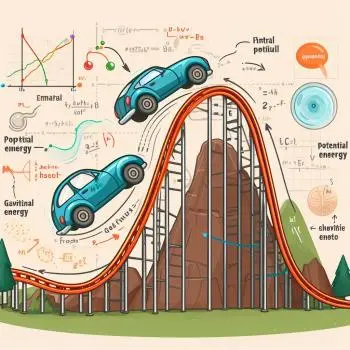# Gravitational Potential EnergyGravitational potential energy, also known as gravity, is a particular type of potential energy that underlies many of the phenomena we experience in our daily lives, from throwing a ball into the air to understanding how hydroelectric plants work.

In this article, we will define gravitational potential energy in detail, the calculation formula with two solved exercises and application examples to help you better understand this type of energy.

## Definition of Gravitational Potential Energy

Gravitational potential energy is a form of energy that an object possesses due to its position in a gravitational field, such as that of the Earth.

When an object is at a certain height above a reference point, such as the ground, it accumulates gravitational potential energy. This energy is ready to be converted into kinetic energy, that is, the energy of motion, when the object falls.

## Formula for Gravitational Potential EnergyThe formula to calculate gravitational potential energy is as follows:

E p =m⋅g⋅h

Where:

• E p is the gravitational potential energy (in joules, J).

• m is the mass of the object (in kilograms, kg).

• g is the acceleration due to gravity at the location where the object is located (in meters per second squared, m/s²).

• h is the height of the object above the reference point (in meters, m).

### Acceleration of Gravity

The value of acceleration due to gravity (g) at the Earth's surface may vary slightly depending on geographic location, altitude, and other local factors, but an average value of approximately 9.81 meters per second squared (m) is commonly accepted. /s²).

## Relationship with the Law of Universal Gravitation

The formula for gravitational potential energy is a direct consequence of Isaac Newton's law of universal gravitation. The relationship between gravitational potential energy and gravitational force is established through the work done by the gravitational force when moving an object in a gravitational field.

When an object moves vertically in a gravitational field (for example, when it falls from a certain height), the work done by the gravitational force is converted to gravitational potential energy.

The relationship between these two formulas is evident when we consider how the work done in moving an object vertically translates into a change in its gravitational potential energy.

## Everyday ExamplesTo better understand this energy concept, we present some real examples:

• A ball in the air: Imagine that you have a ball in your hand and you raise it to a certain height above the ground. In this case, the gravitational potential energy of the ball increases as you raise it. When you release it, the potential energy is converted to kinetic energy, and the ball falls toward the ground.

• Hydroelectric power plant: Hydroelectric power plants take advantage of the gravitational potential energy of water stored in an elevated reservoir. When a floodgate opens, water falls from a great height, spinning turbines and generating electricity in the process.

• Parachute jump: When a skydiver ascends in an airplane, he accumulates gravitational potential energy. When jumping, potential energy is converted into kinetic energy while performing what in kinematics is known as a uniformly accelerated linear movement.

• Projectile Launch: In projectile launching situations, such as a space rocket, gravitational potential energy is converted to kinetic energy as the object ascends. As the projectile ascends, its potential energy increases, and as it descends, this potential energy is converted into kinetic energy due to the law of conservation of energy.

## Solved Exercises

Now let's look at some examples of solved exercises in which the formula shown above is applied:

### Exercise 1

Calculate the gravitational potential energy of a rock with a mass of 5 kg that is at a height of 10 meters above the ground. It uses an acceleration due to gravity of 9.81 m/s².

Solution

E p =m⋅g⋅h

E p = 5kg ⋅ 9.81m/s² ⋅ 10m = 490.5J

The gravitational potential energy of the rock is 490.5 joules.

### Exercise 2

Suppose a person lifts a 20 kg box to a height of 2 meters above the ground. Calculate the gravitational potential energy of the box.

Solution

E p =m⋅g⋅h

E p   = 20kg ⋅ 9.81m/s² ⋅ 2m = 392.4J

The gravitational potential energy of the box is 392.4 joules.

Author:

Published: October 20, 2023
Last review: October 20, 2023